# CHSE Odisha Class 12 Math Solutions Chapter 7 Continuity and Differentiability Ex 7(k)

Odisha State Board Elements of Mathematics Class 12 CHSE Odisha Solutions Chapter 7 Continuity and Differentiability Ex 7(k) Textbook Exercise Questions and Answers.

## CHSE Odisha Class 12 Math Solutions Chapter 7 Continuity and Differentiability Exercise 7(k)

Question 1.
State True (T) or False (F).
(i) There is no function whose derivative is log π.
Solution:
False

(ii) There is no function which is its own derivative.
Solution:
False

(iii) A function is not differentiable at x = c ⇒ f is not continuous at x = c.
Solution:
False

(iv) |x2| is differentiable on (- 1, 1).
Solution:
True

(v) |x + 2| is not differentiable at x = 2.
Solution:
False

(vi) Derivative of e3 log x w.r.t. x is 3x2.
Solution:
True

(vii) The derivative of a non constant even function is always an odd function.
Solution:
True

(viii) If f and g are not derivable at x0 then f + g is not derivable at x0.
Solution:
FalseQuestion 2.
Fill up the gaps by using the correct answer.
(i) If a is a constant and v is a variable then $$\frac{d u^v}{d v}$$ = _______. (uv In v, vuv – 1, uv In u, uvv – 1)
Solution:
uv In v

(ii) If t = ea then $$\frac{d}{d x}$$xt = _______. (txt – 1, xt, xt In a, txt)
Solution:
txt – 1

(iii) If u = t2 and v= sin t2 then $$\frac{d v}{d u}$$ = _______. (cos2 t, $$\frac{\sin }{t}$$, sec t2, cos t2)
Solution:
cos t2

(iv) The tangent to the curve y = (1 + x2)2 at x = -1 has slope _______. (4, -4, 8, -8)
Solution:
-8

(v) If v = (gof) (x) then $$\frac{d y}{d x}$$ = _______. ($$\frac{d g}{d x} \frac{d x}{d f}$$, $$\frac{d g}{d f} \frac{d f}{d x}$$, $$\frac{d f}{d x} \frac{d x}{d g}$$, $$\frac{d f}{d g} \frac{d g}{d x}$$)
Solution:
$$\frac{d g}{d f}\frac{d f}{d x}$$

(vi) If y = sec-1 $$\frac{\sqrt{x}+1}{\sqrt{x}}$$ + $$\frac{\sqrt{x}}{\sqrt{x}+1}$$ then $$\frac{d y}{d x}$$ = _______. (0, undefined, $$\frac{\pi}{2}$$, 1)
Solution:
0

(vii) If (x) = $$\sqrt{x^2-2 x+1}$$, x ∈ [0, 2] then at x = 1, f(x) = _______. (1, 0, -1, does not exist)
Solution:
does not exist

(viii) If f(x) = |x2| then f'($$\frac{3}{2}$$) = _______. (0, 2, 3, does not exist)
Solution:
0

Question 3.
Differentiate from first principles.
(i) e2x
Solution:
Let y = e2x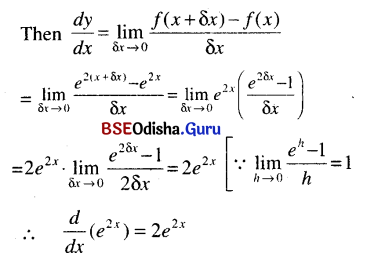(ii) sin2 x
Solution:
Let y = sin2 x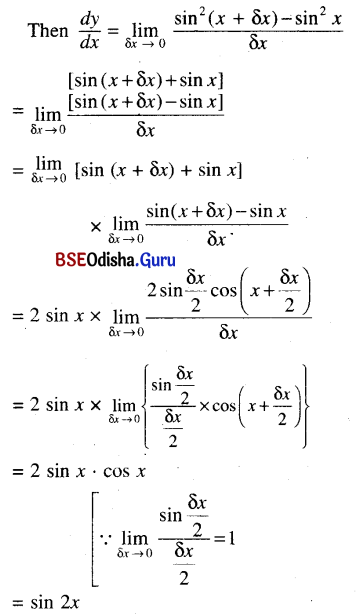(iii) cos x2
Solution:
Let y = cos x2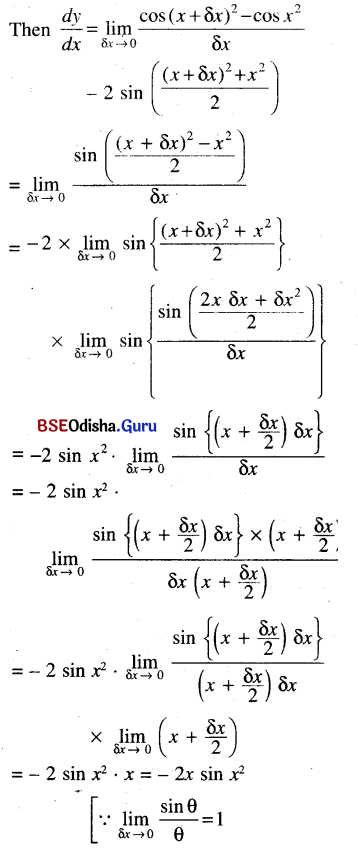(iv) $$\boldsymbol{e}^{x^2}$$
Solution: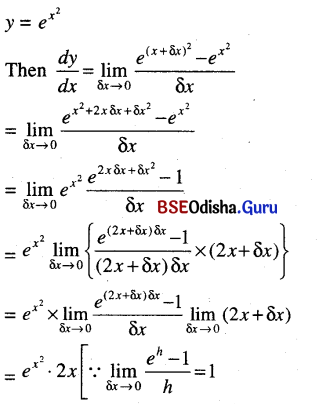(v) $$\sqrt{\tan x}$$
Solution: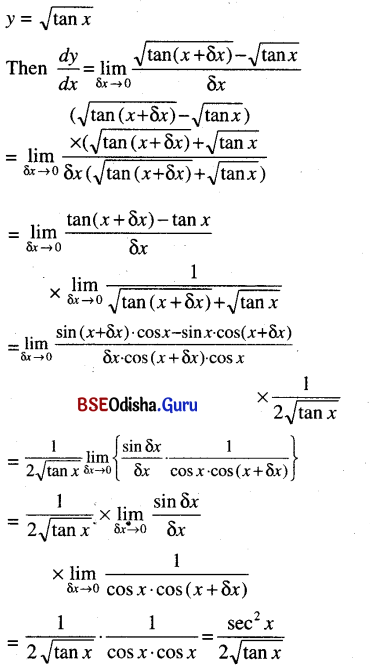(vi) x2 sin x
Solution:
Let y = x2 sin x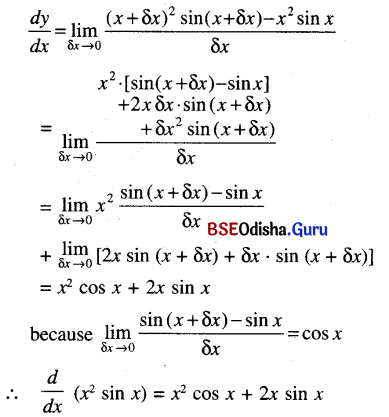(vii) In sin x
Solution:
Let y = In sin x
Then ey = sin x  … (1)
Let dx be an increment of x and δv be the corresponding increment of y.
Then ey + δy = sin (x + δx)  … (2)
Subtracting (1) from (2) we get,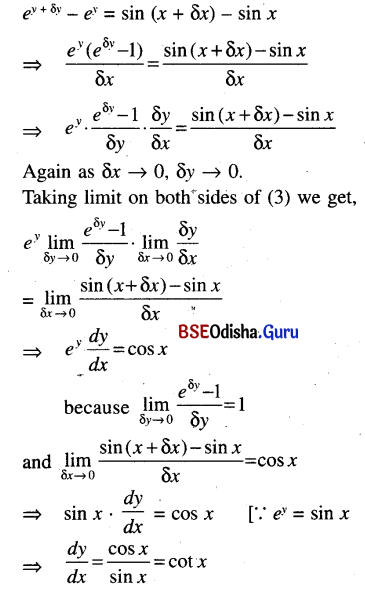(viii) sin √x
Solution:
y = sin √x
Put u = √x
Then y = sin u
Let δx be an increment of x and δu, δy be the corresponding increment of u and y respectively.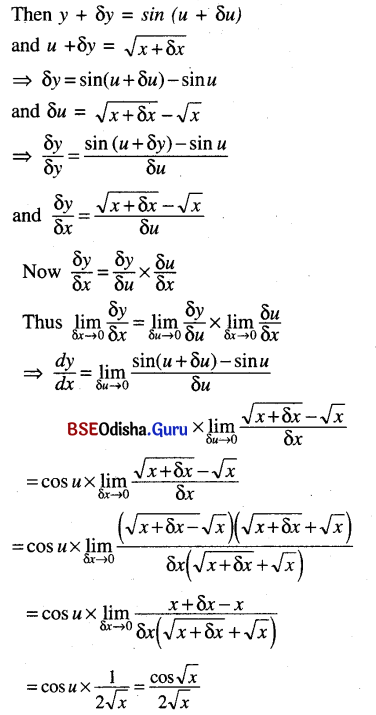(ix) cos In x
Solution:
Let y = cos (In x)
Let u = In x
Then y = cos u
Suppose that δx be an increment of x and δu, δy be corresponding increments of u and y respectively.
Then y + δy = cos (u + δu)  ….(3)
and u + δu = In (x + δx)  ….(4)
Subtracting (2) from (3) and (1) from (4) we get
δy = cos (u + δu) – cos u
and δu = In (x + δx) – In x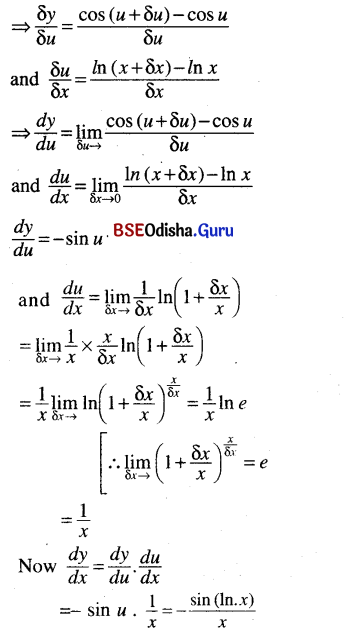Question 4.
Test differentiability of the following functions at the indicated points.
(i) f(x) =[x2 + 1] at x = –$$\frac{1}{2}$$
Solution: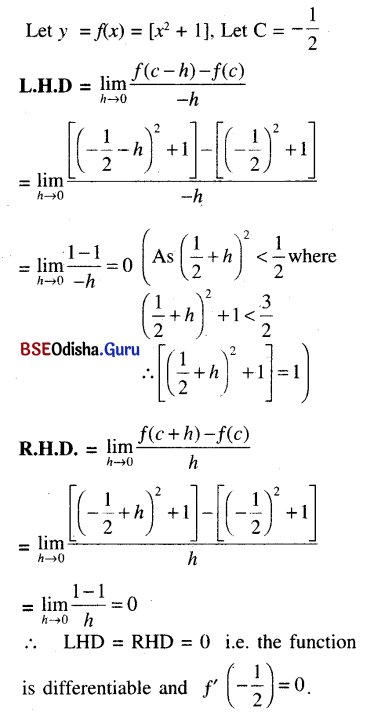(ii) f(x) = $$\begin{cases}1-2 x, & x \leq \frac{1}{2} \\ x-\frac{1}{2}, & x>\frac{1}{2}\end{cases}$$ at x = $$\frac{1}{2}$$
Solution: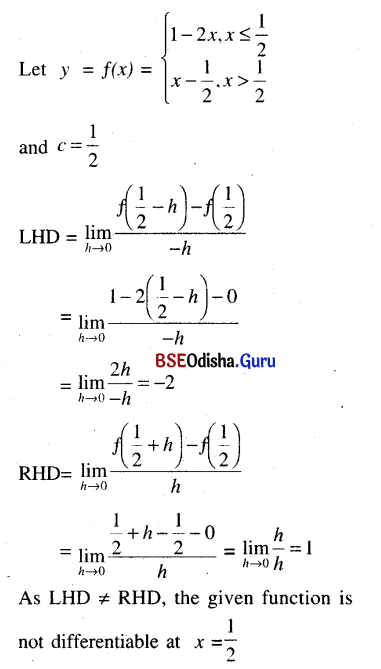(iii) f(x) = x + |cos x| at x = $$\frac{\pi}{2}$$
Solution:
f(x) = x + |cos x| at c = $$\frac{\pi}{2}$$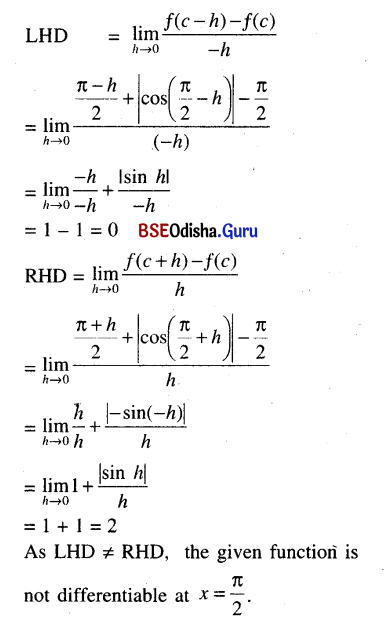Hence onwards domain of a function is to be understood to be its natural domain unless stated otherwise.

Question 5.
Differentiate.
(i) $$\frac{1}{\ln (x \sqrt{x+1})}$$
Solution: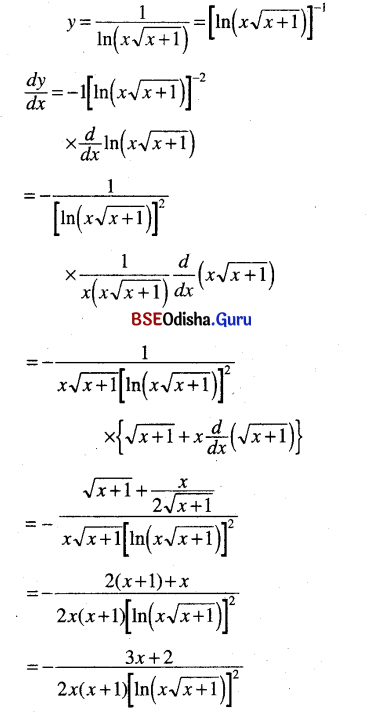(ii) $$\frac{\ln x}{e^x \sin x}$$
Solution: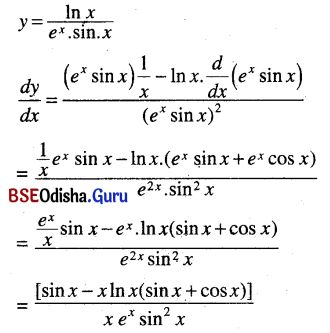(iii) ex (tan x – cot x)
Solution:
y = ex (tan x – cot x)
$$\frac{d y}{d x}$$ = $$\frac{d}{d x}$$ (ex) (tan x – cot x) + ex $$\frac{d}{d x}$$ (tan x – cot x)
= ex (tan x – cot x) + ex (sec2 x + cosec2 x)

(iv) $$\left(\sqrt{x}+\frac{1}{\sqrt{x}}\right)$$x tan x
Solution: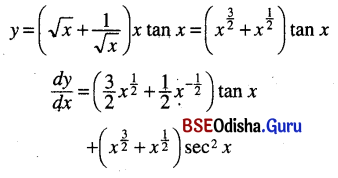(v) $$\frac{\cos 3 x-\cos x}{\cos 5 x-\cos 3 x}$$
Solution: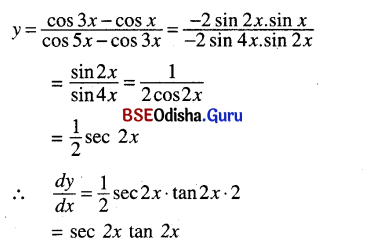(vi) x2 ex cosec x
Solution:
y = x2 ex cosec x
$$\frac{d y}{d x}$$ = $$\frac{d}{d x}$$ (x2) ex . cosec x + x2 $$\frac{d}{d x}$$ (ex) . cosec x + x2 ex $$\frac{d}{d x}$$
= 2x ex cosec x + x2 ex cosec x – x2 ex . cosec x . cot x

(vii) $$\frac{(x+1) \ln x}{\sqrt{x+2}}$$
Solution: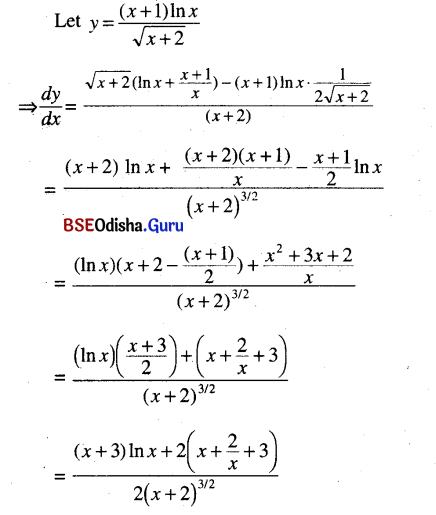(viii) (x3 – 1)9 sec2 x
Solution: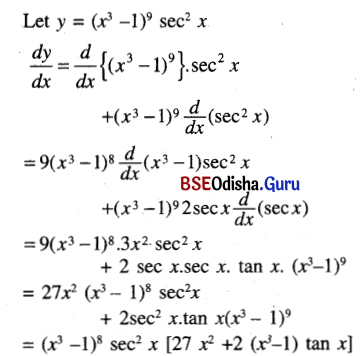(ix) sin2 (cos-1 x)
Solution: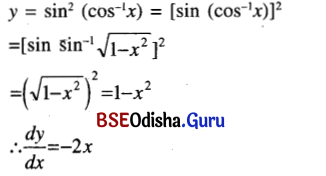(x) ax $$\left(x+\frac{1}{x}\right)^{10}$$
Solution: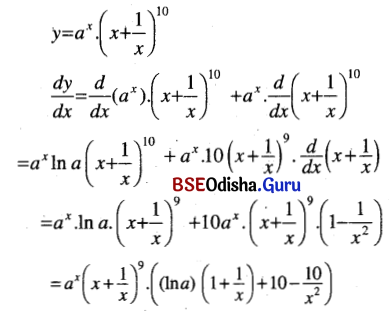(xi) In $$\frac{\sqrt{x+4}-2}{\sqrt{x+4}+2}$$
Solution: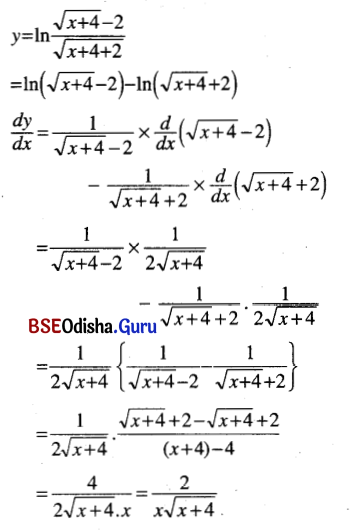(xii) In $$\frac{4 x^2(2 x-7)^3}{\left(3 x^2-7\right)^5}$$
Solution: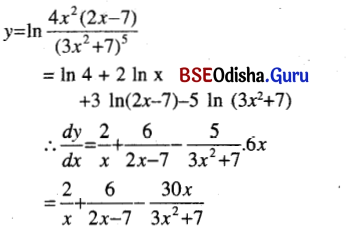(xiii) 5ln sin x
Solution: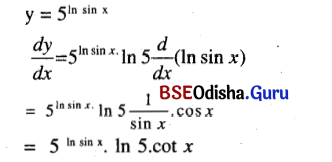(xiv) $$\sqrt{\sin \sqrt{x}}$$
Solution: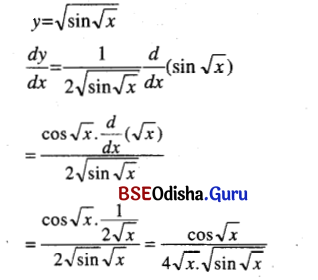(xv) xsin x + (tan x)x
Solution:
Let y = xsin x + (tan x)x
Put u = xsin x , v = (tan x)x
Then y = u + v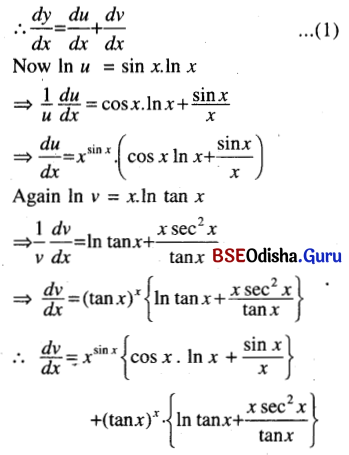(xvi) $$e^{e^x}$$
Solution: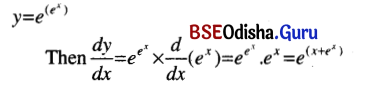(xvii) $$x^{\sqrt{x}}$$
Solution: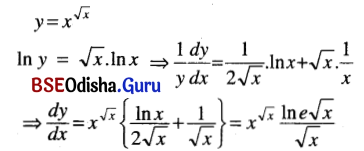(xviii) sec-1(ex + x)
Solution: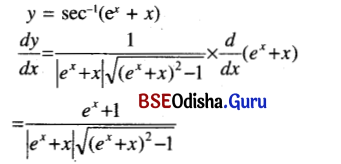(xix) ln cos ex
Solution: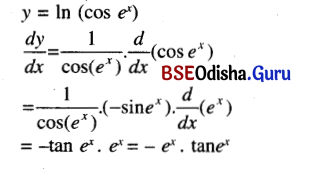(xx) $$a^{\sin ^{-1} x^2}$$
Solution: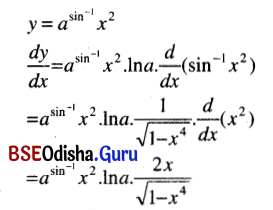(xxi) cos-1 $$\left(\frac{x^4-1}{x^4+1}\right)$$
Solution: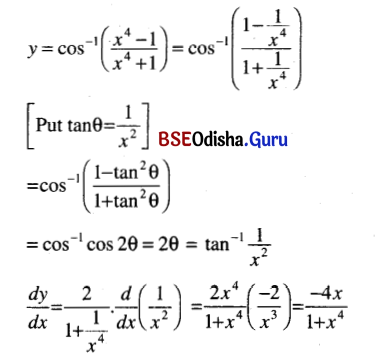(xxii) $$\left(\mathbf{x}^{\mathbf{e}}\right)^{\mathbf{e}^{\mathrm{x}}}$$ + $$\left(\mathrm{e}^{\mathrm{x}}\right)^{\mathrm{x}^e}$$
Solution: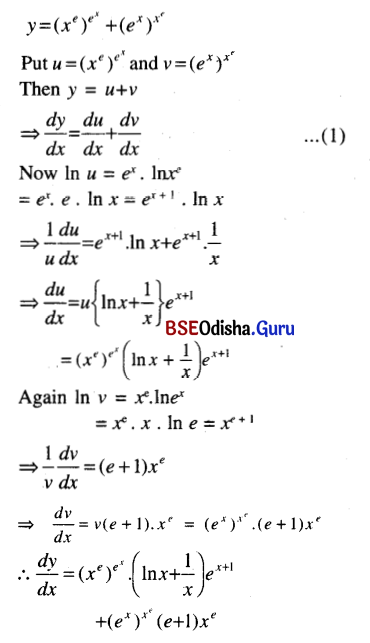(xxiii) $$\boldsymbol{x}^{\left(\boldsymbol{x}^x\right)}$$
Solution: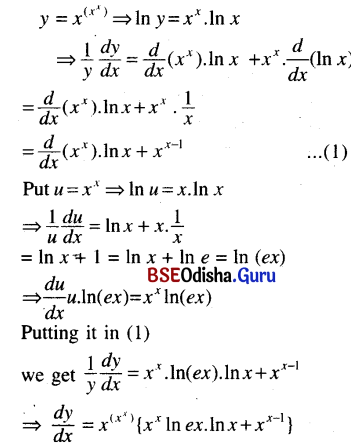(xxiv) $$\frac{\left(x+1^2\right) \sqrt{x-1}}{\left(x^2+3\right)^3 3^x}$$
Solution: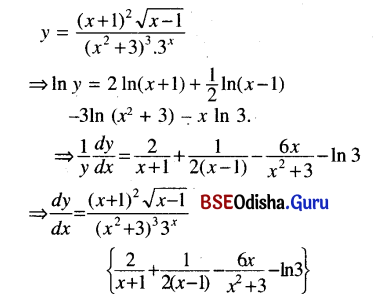(xxv) [5 In (x3 + 1) – x4]2/3
Solution: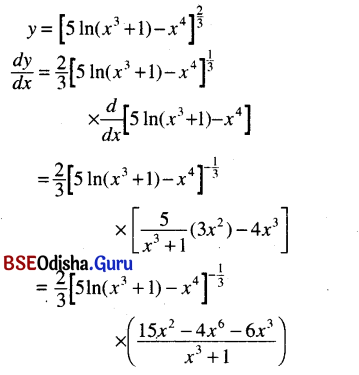(xxvi) log10 sin x + logx 10, 0 < x > π.
Solution: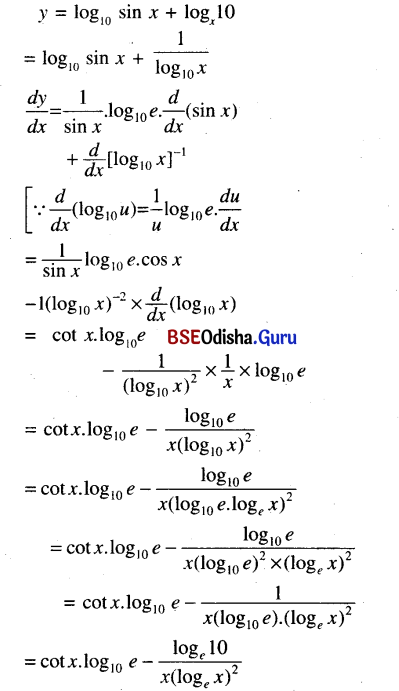Question 6.
Differentiate
(i) sec-1 $$\left(\frac{x^2+1}{x^2-1}\right)$$
Solution: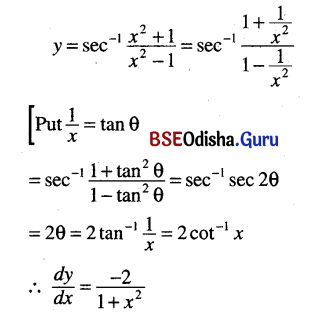(ii) $$e^{\tan ^{-1} x^2}$$
Solution: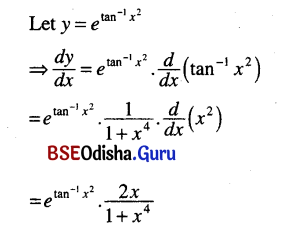(iii) $$\frac{x \sin ^{-1} x}{\sqrt{1+x^2}}$$
Solution: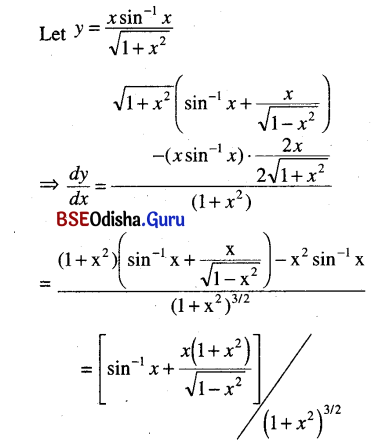(iv) tan-1 e2x
Solution: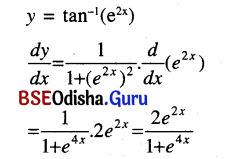(v) tan-1 $$\frac{\cos x}{1+\sin x}$$
Solution: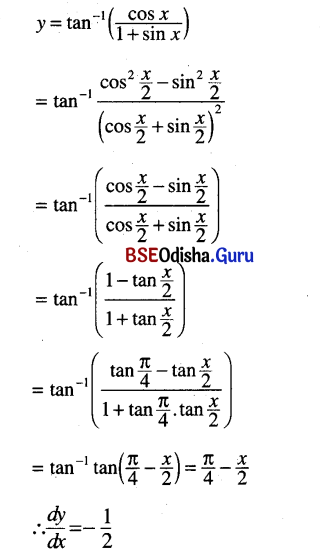(vi) tan-1 $$\left(\frac{\cos x-\sin x}{\cos x+\sin x}\right)$$
Solution: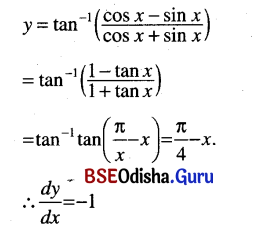(vii) tan-1 $$\frac{7 a x}{a^2-12 x^2}$$
Solution: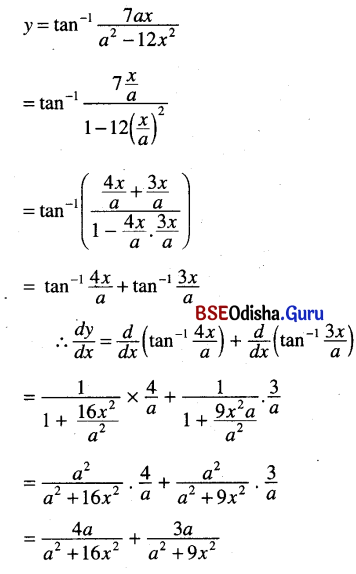(viii) tan-1 $$\frac{\sqrt{1+x^2}+\sqrt{1-x^2}}{\sqrt{1+x^2}-\sqrt{1-x^2}}$$ (Put x2 = cos θ)
Solution: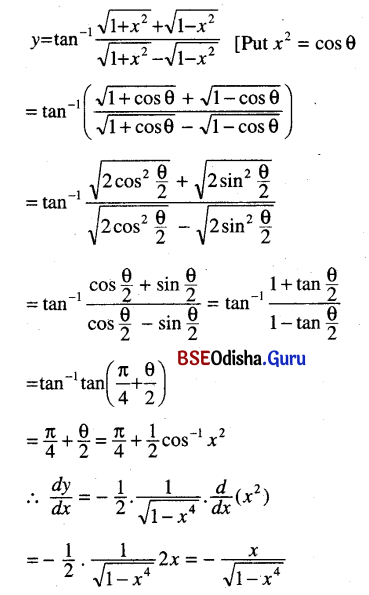(ix) x2 cos $$\frac{\sqrt{x}-1}{\sqrt{x}+1}$$ = x2 cosec-1 $$\frac{\sqrt{x}+1}{\sqrt{x}-1}$$
Solution: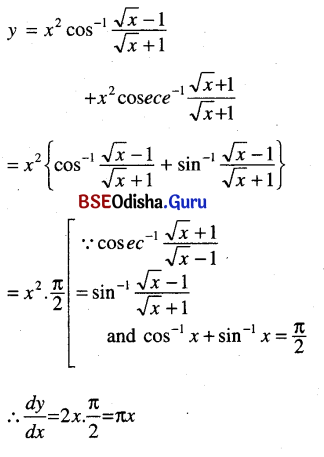(x) tan-1 $$\frac{x}{1+\sqrt{1-x^2}}$$
Solution: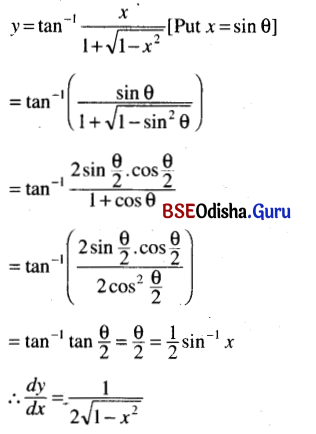(xi) tan-1 $$\left(\frac{x \sin \alpha}{1-x \cos \alpha}\right)$$
Solution: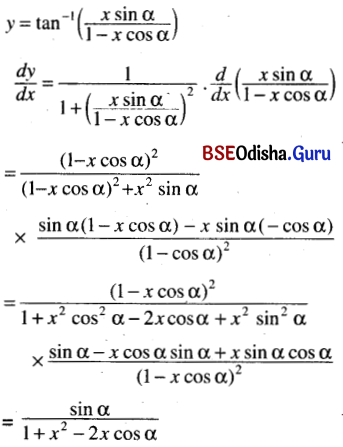Question 7.
Find $$\frac{d y}{d x}$$ if
(i) x3 + y3 = 12xy
Solution: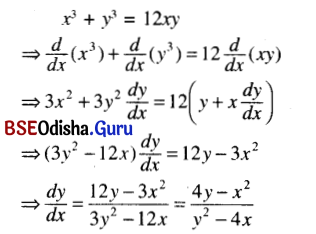(ii) $$\left(\frac{x}{a}\right)^{2 / 3}$$ + $$\left(\frac{y}{b}\right)^{2 / 3}$$ = 1
Solution: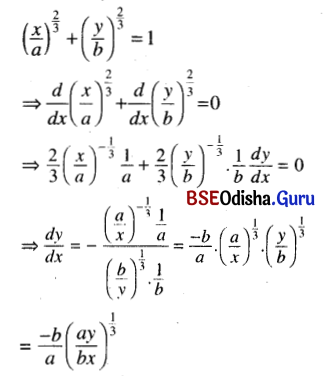(iii) xy = c
Solution: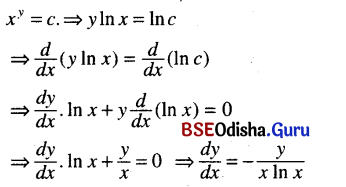(iv) yx = c
Solution:
yx = c ⇒ x In y = In c
⇒ in y + $$\frac{x}{y} \frac{d y}{d x}$$ = 0
⇒ $$\frac{d y}{d x}$$ = –$$\frac{y \ln y}{x}$$

(v) x cot y + cosec x = 0
Solution: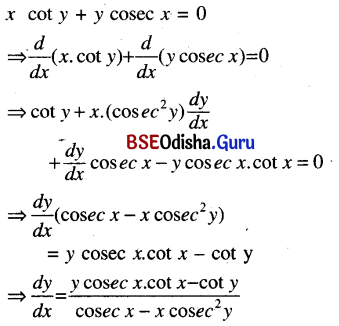(vi) y2 + x2 = In (xy) + 1
Solution: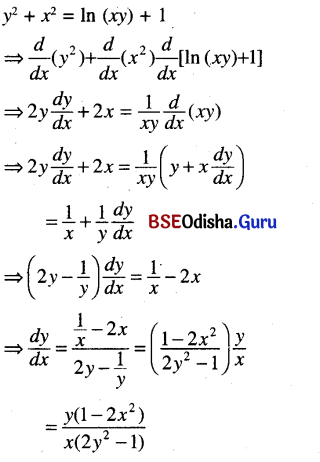(vii) (cos x)y = sin y
Solution: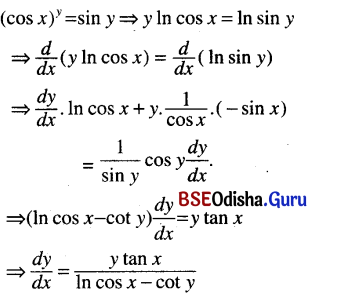(viii) y2 =a√x
Solution: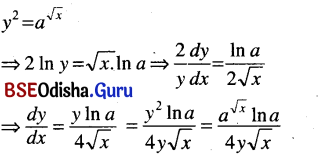(ix) xm yn = $$\left(\frac{x}{y}\right)^{m+n}$$
Solution: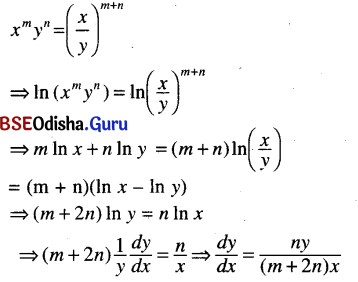(x) y = x cot -1 $$\left(\frac{x}{y}\right)$$
Solution: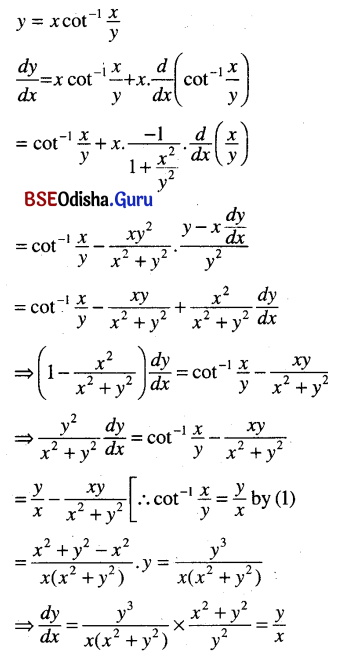(xi) y = (sin y)sin 2x
Solution: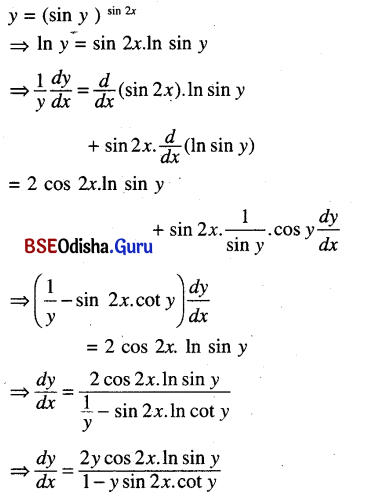(xii) y2 = xy
Solution: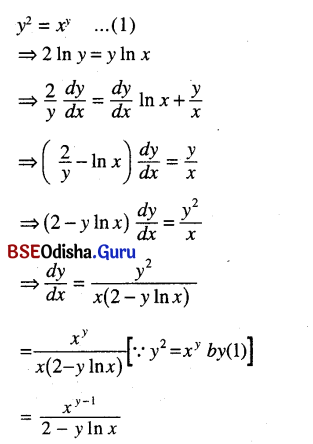(xiii) (x + y)cos x = e x + y
Solution: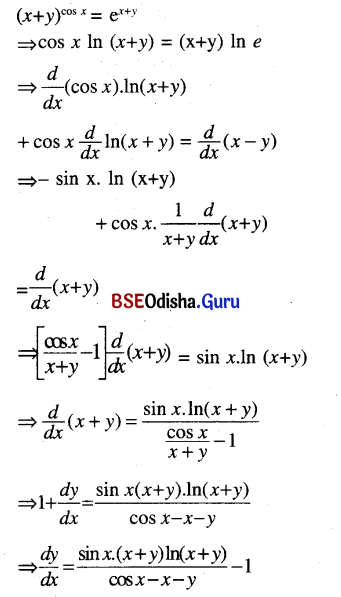(xiv) x tan y + y tan x = 0
Solution:(xv) $$\sqrt{x^2+y^2}$$ k tan-1 $$\left(\frac{y}{x}\right)$$
Solution: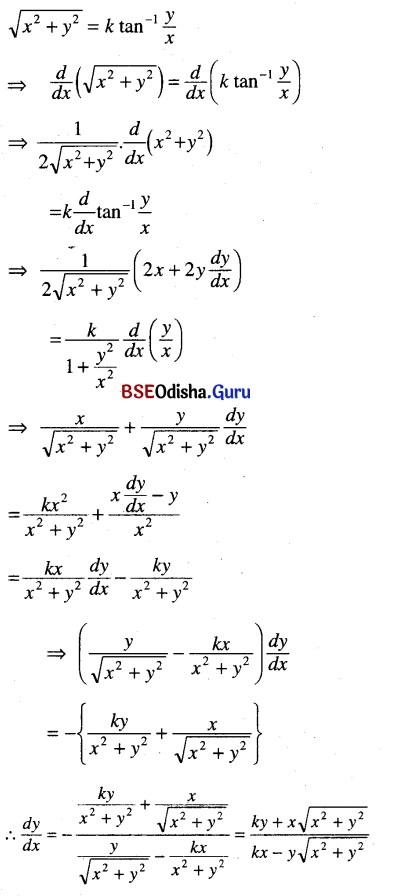Question 8.
Differentiate
(i) tan-1 $$\frac{2}{1-x^2}$$ w.r.t. sin-1 $$\frac{2}{1+x^2}$$
Solution: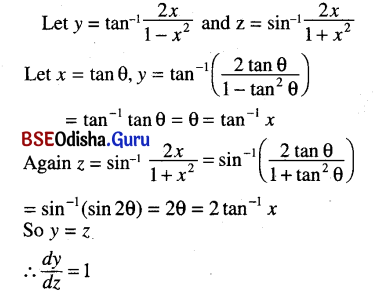(ii) sec-1 $$\left(\frac{1}{2 x^2-1}\right)$$ w.r.t. $$\sqrt{1-x^2}$$
Solution: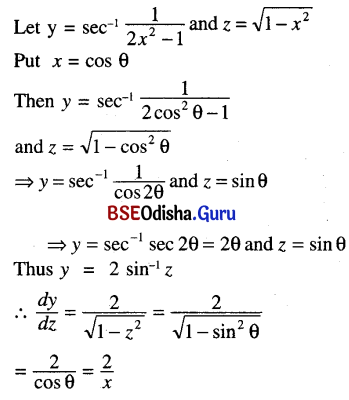(iii) tan-1 $$\left(\frac{1+\sin x}{1-\sin x}\right)$$ w.r.t. log $$\left(\frac{1+\cos x}{1-\cos x}\right)$$
Solution: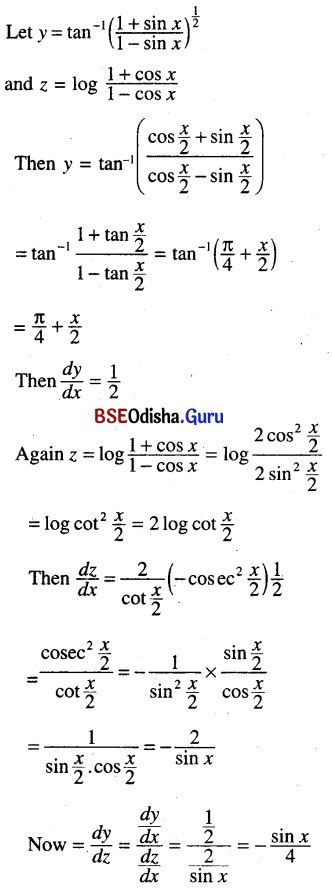Question 9.
Find the $$\frac{d y}{d x}$$ when
(i) x = a [cos t + log tan ( t/2)], y = a sin t
Solution: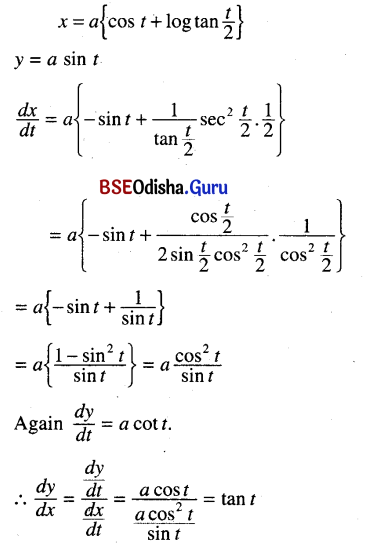(ii) sin x = $$\frac{2 t}{1+t^2}$$, tan y = $$\frac{2 t}{1-t^2}$$
Solution: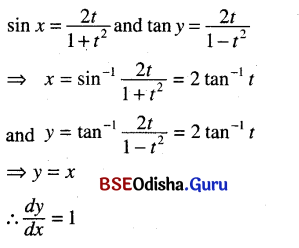(iii) cos x= $$\sqrt{\frac{1}{1+t^2}}$$, siny = $$\frac{2 t}{1+t^2}$$
Solution: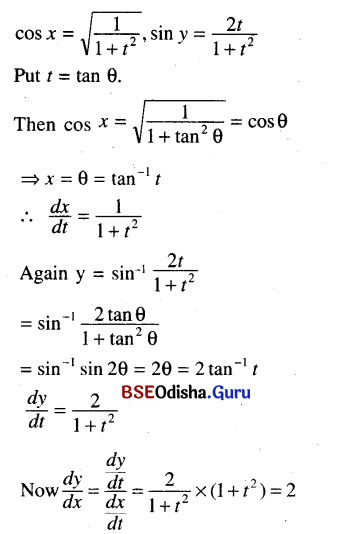(iv) cos x = $$\sqrt{\sin 2 u}$$, y = $$\sqrt{\cos 2 u}$$
Solution: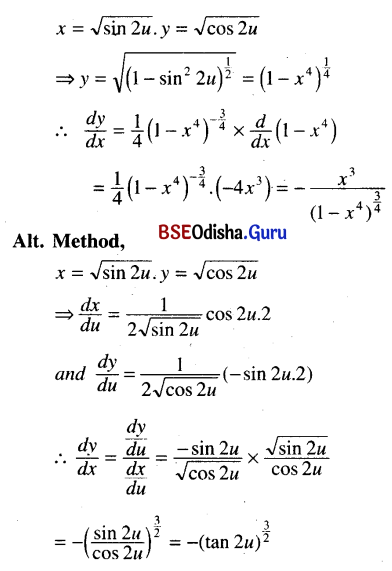(v) x = $$\frac{\cos ^3 t}{\sqrt{\cos 2 t}}$$, y = $$\frac{\sin ^3 t}{\sqrt{\cos 2 t}}$$
Solution: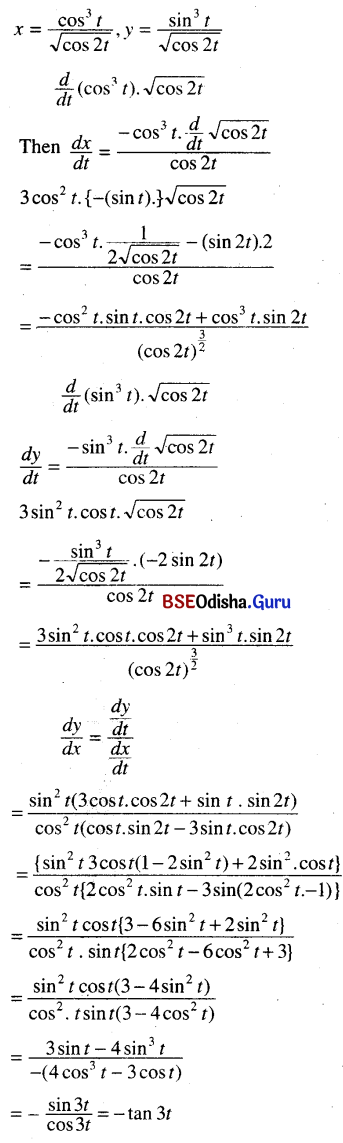Question 10.
Assuming the validity of the operations on the r.h.s. find $$\frac{d y}{d x}$$.
(i) y = [ sin x + { sin x + (sin x +….)}]
Solution: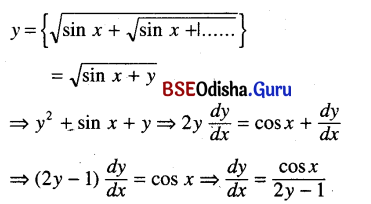(ii) y = 1 ÷ [ x + 1 ÷ (x + 1 ÷ (x + 1 ÷ …))]
Solution: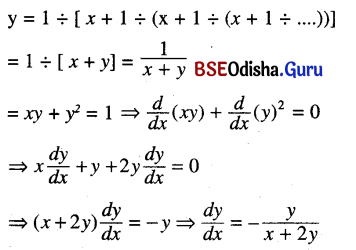(iii) y = In [x + In (x + In (x + ….))]
Solution: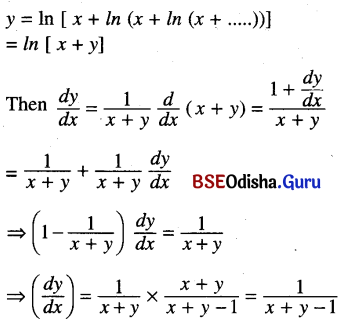Question 11.
If cos y = x cos (a + y) then prove that
(i) $$\frac{d y}{d t}$$ = $$\frac{\cos ^2(a+y)}{\sin a}$$
Solution: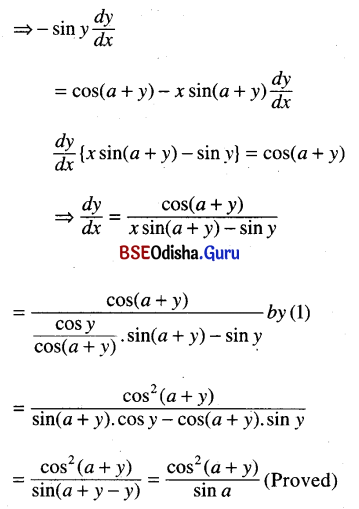(ii) If eθΦ = c + 4 θΦ , show that Φ + θ $$\frac{d \phi}{d \theta}$$ = 0.
Solution: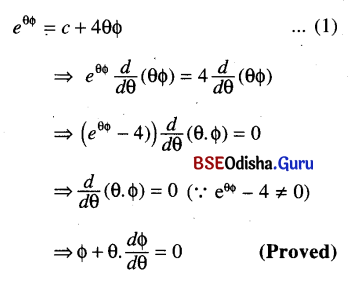Question 12.
Solution:
Clearly for all x ∈ R
sin x ∈ [- 1, 1]
⇒ |sin x| ∈ [ 0,1]
⇒ log |sin x| ∈ (-∞ , 0]
⇒ log log |sin x| is not well defined for all x ∈ R
∴ Log log |sin x| is not a differentiable function.

Question 13.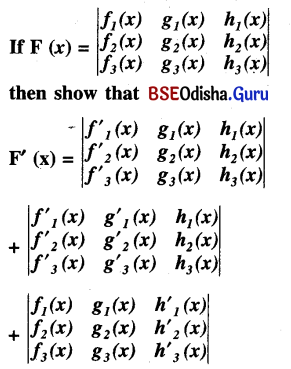Solution: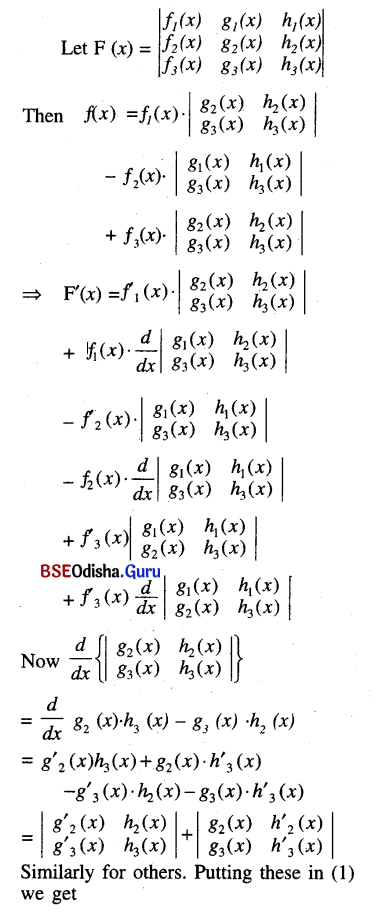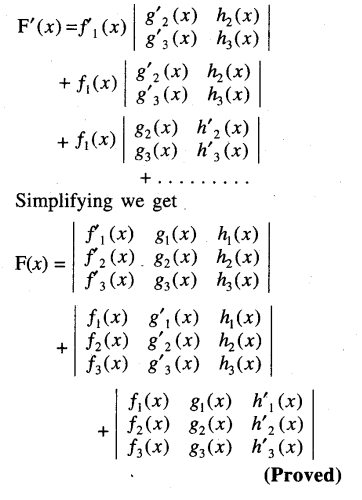Question 14.
If x = $$\frac{1-\cos ^2 \theta}{\cos \theta}$$, y = $$\frac{1-\cos ^{2 n} \theta}{\cos ^n \theta}$$ then show that $$\left(\frac{d y}{d x}\right)^2$$ = n2$$\left(\frac{y^2+4}{x^2+4}\right)$$
Solution: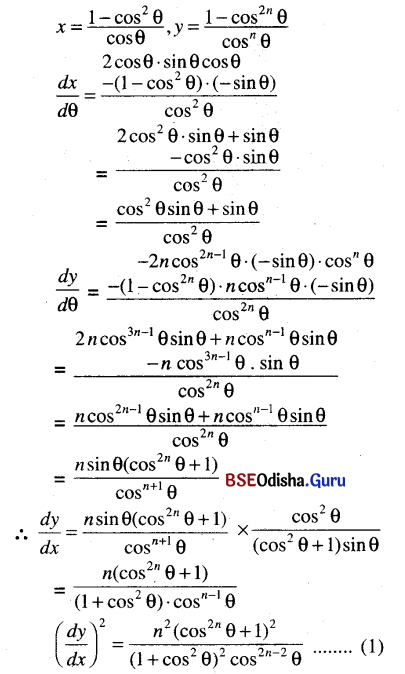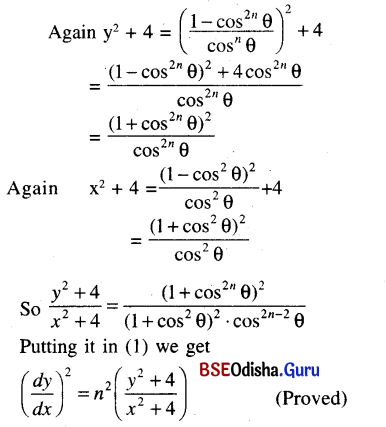Question 15.
Show the $$\frac{d y}{d x}$$ is independent of t if.
x = cos-1$$\frac{1}{\sqrt{t^2+1}}$$, y = sin-1$$\frac{t}{\sqrt{t^2+1}}$$
Solution: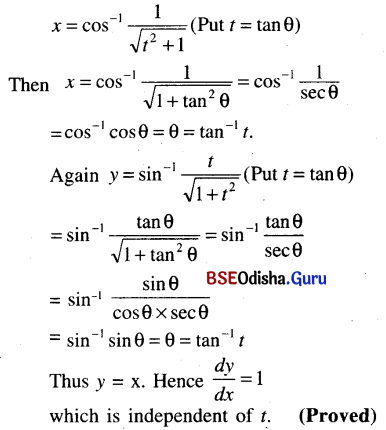Question 16.
If y $$\sqrt{x^2+1}$$ = {$$\sqrt{x^2+1}$$ – x} then prove that (x2 + 1) $$\frac{d y}{d x}$$ + xy + 1 = 0
Solution: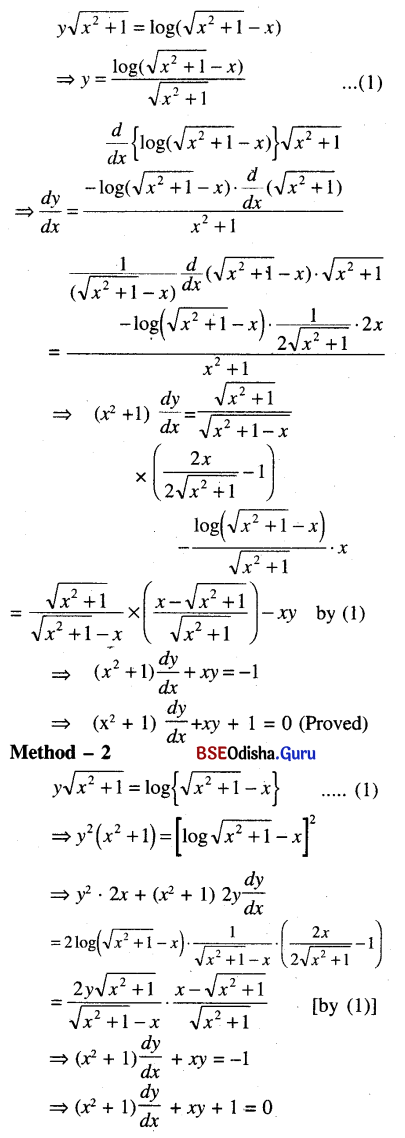Question 17.
If ey/x = $$\frac{x}{a+b x}$$, then show that x3 $$\frac{d}{d x}$$ $$\left(\frac{d y}{d x}\right)$$ = $$\left(x \frac{d y}{d x}-y\right)^2$$
Solution: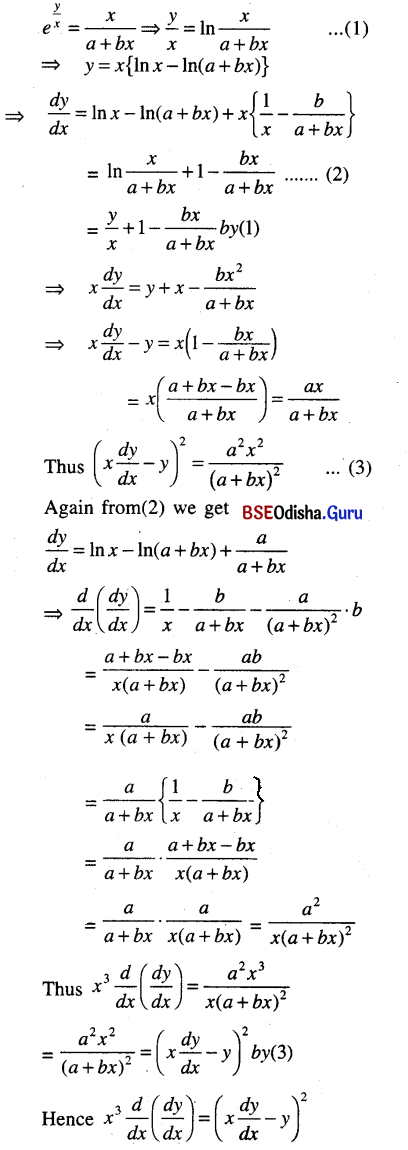Question 18.
Find the points where the following functions are not differentiable.
(i) e|x|
Solution:
e|x| is not differentiable at x = 0 because |x| is not differentiable at x = 0

(ii) |x2 – 4|
Solution:
|x2 – 4| is not differentiable at the points where x2 – 4 = 0 i.e, x =± 2.

(iii) |x – 1| + |x – 2|
Solution:
|x – 1| + |x – 2| is not differentiable at x = 1 and x = 2.

(iv) sin |x|
Solution:
sin |x| is not differentiable at x = 0.